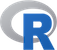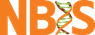@# Introduction

There is a number of R functions available in the basic, pre-loaded packages, such as base, utils or stats and there is a huge number of functions available via third-party packages. Today, we will work a bit with some of the functions that are commonly used by R users coming from different disciplines. In particular, we will:

• Learn how to work with sets.
• Work with polynomials.
• Learn how to define and work with functions.
• Do some basic calculus.
• Learn about formulas.
• Work with some selected statistical tests.
• Learn more about setting up a statistical analyses workflow by fitting a linear model to example data.

## Exercise 1. Create and work with sets

1. Open R-studio and create three sets of genes:
G1 <- {‘ANK-1’, ‘ANK-c’, ‘GALNTL-1’}
G2 <- {‘ANK-1’, ‘FMA’, ‘RHO’, ‘GRP’}
G3 <- {‘GALNTL-1’, ‘ANK-c’, ‘HQX’}.
Visualise membership relations between all elements of the sets using Venn diagram. Use package venn.Click to see how
 library('venn')
G1 <- c('ANK-1', 'ANK-c', 'GALNTL-1')
G2 <- c('ANK-1', 'FMA', 'RHO', 'GRP')
G3 <- c('GALNTL-1', 'ANK-c', 'HQX')
venn(list(G1 = G1, G2 = G2, G3 = G3))


2. What is the:
• Union of G1 and G3?
• Union of intersections: G1 with G2 and G1 with G3?
• Difference between G2 and G3?
• Is union of G1 and G2 equal to the intersection of G2 with G3?
• Are genes ANK-c and GALNTL-1 members of the intersection of G1 with G3?Click to see how
 union(G1, G3)
union(intersect(G1, G2), intersect(G2, G3))
setdiff(G2, G3)
setequal(union(G1, G2), intersect(G2, G3))
is.element(c('ANK-c', 'GALNTL-1'), intersect(G1, G3))


## Exercise 2. Define and work with polynomials

1. Define the following polynomials p1 and p2: 5x^3 + 4x^2 + 7 and 2x^2 + 3x - 11.Click to see how
 library(polynom)
p1 <- polynomial(c(7, 0, 4, 5))
p2 <- polynomial(c(-11, 3, 2))


2. Define a polynomial (p3) with the following zeros: -3, 4, 7.Click to see how
 library(polynom)
p3 <- poly.calc(c(-3, 4, 7))


3. Define a polynomial (p4) passing through the following points: A(-3,7), B(24,-9), C(7,4).Click to see how
 p4 <- poly.calc(c(-3, 24, 7), c(7, -9, 4))


4. Find approximate maximum (value) of the p4 polynomial using its visualisation.Click to see how
 plot(p4, ylim=c(-1, 8))
#The maximum is between 6 and 8.


5. Perform the following operations:
• Find the sum of p1 and p3.
• Divide p4 by p2.
• Find the area under p4 on the [-10, 0] interval.
• Find the second order derivative of p1.
• Find the Greatest Common Divisor of p1 and p2.
• Find the Least Common Multiple of p1 and the sum of p2 and p3.Click to see how
 p1 + p3
p4 / p2
integral(p4, c(-10, 0))
deriv(deriv(p1))
GCD(p1, p2)
LCM(p1, p2 + p3)


## Exercise 3. Formulas

Observe that there is a convenient way of validating your formulas:

# First, define the formula
frm <- formula(y ~ (v1 + v2 + v3) %in% v)
# Check the validity of the above
terms <- attr(terms.formula(frm), "term.labels")
# See all terms that will be added together
terms
# Here: y ~ v1:v + v2:v + v3:v


Write formulas describing the following relations of y:

• depends on var1 and var2 but not on their interaction,
• depends on height but without the constant term,
• depends on interactions of ‘a’ with ‘b’ and ‘a’ with ‘c’ and ‘a’
with ‘d’ but without additive effects,
• like above, but with additive effects of single variables,
• like above, but without the constant term and without the additive
effect of ‘c’.Click to see how
	y ~ var1 + var2
y ~ +0 + height
frm <- formula(y ~ (b + c + d) %in% a)
#Check the validity of the above
terms <- attr(terms.formula(frm), "term.labels")
y ~ a * (b + c + d)
y ~ a * (b + c + d) - c


## Exercise 4. Define and work with functions

1. Define function: f(x) = 2x^3 + 3x^2 + sin(x/2) + 7 and finds its value for x = 42.Click to see how
 f <- function(x) {
y = 2*x^3 + 3*x^2 + sin(x/2) + 7
return(y)
}
f(42)

f(42) = 153476


2. Find the f(x) zeros on the [-10, 10] interval, values at zeros and plot the function for the same interval.Click to see how
 uniroot(f, lower=-10, upper=10)
curve(f, from=-10, to=10)

One zero: $f(-2.16) = 5.24\times10^{-5}$


3. Find the first order derivative of f(x) with respect to x. Use both D() and deriv(). Are the resulting derivatives the same? What is the difference between the functions?Click to see how
 D(expression(2*x^3 + 3*x^2 + sin(x/2) + 7), name='x')
deriv(~2*x^3 + 3*x^2 + sin(x/2) + 7, 'x')

The resulting derivatives are the same, just written in different
ways. *D()* takes an expression as argument and it returns an
expression while *deriv()* works on formulas.


4. Evaluate the derivative from FNS4.3 at x = {1, 3, 7}. What values does it take at these points?Click to see how
 my.call <- D(expression(2*x^3 + 3*x^2 + sin(x/2) + 7), name='x')
x <- c(1, 3, 7)
eval(my.call)

  12.4  72.0 335.5



5. Perform numerical integration: \int_1^7 f(x).
• What is the value?
• What is the absolute estimation error?Click to see how
 integrate(f, lower = 1, upper = 7)

1588 with absolute error < 1.8e-11


## Exercise 5. Statistical tests

Draw N=30 random observations from N(0,1) (normal distribution with mean=0 and std. dev.=1) and N=38 random observations from N(0.02,1.1).

• What statistical test(s) would you use to check whether the two samples come from the normal distribution?
• Can we really say something about the samples’ normality? Why?
• What parametric test would you use to check whether both samples come from the distribution with the same mean?
• What do you have to check before you apply the test?Click to see the answer - For example, one can plot a QQ plot for both samples. One can also use Shapiro-Wilk test for normality. Can you think of any more tests? - Well, here sample size is low, we may get false results! - For instance the Student's t-test. It is appropriate for sample sizes below N=100. As a rule of thumb, N=30 is about sufficient. - Before applying the test, one has to check whether its assumptions are valid. Here, we have to check the normality first.

## Exercise 6. Linear model and diagnostics

Package UsingR contains a data with fat measurements in 252 males.

• load the dataset (install the package if necessary),
• read about the dataset in package help,
• construct a linear model with body.fat as the dependent variable and variables: age, weight, height, neck and hip as independent variables,
• perform model diagnostics,
• improve the model if necessary (hint: e.g. outliers),
• which variables are significant?
• how much of the variability can be explained by the model?
• how would you improve the model? Do this if you have time,Click to see how
library('UsingR')
data('fat')
?fat
model <- lm(body.fat ~ age + weight + height + neck + hip,  data=fat)
summary(model)
plot(model)
#Remove outliers
fat2 <- fat[-c(36, 39, 42, 216), ]
model2 <- lm(body.fat ~ age + weight + height + neck + hip,  data=fat2)
summary(model2)
plot(model2)